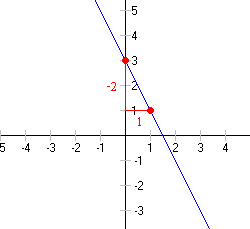# Slope-Intercept Form

If you know the slope $m$ , and $y$ -intercept $\left(0,b\right)$ of a line (the point where the line crosses the $y$ -axis), you can write the equation of the line in slope-intercept form .

$y=mx+b$

(You can think of this as a special case of the point-slope form of the equation where $\left({x}_{1},{y}_{1}\right)$ is the point $\left(0,b\right)$ .)

Example 1 :

Find an equation of the line in slope-intercept form with slope $3$ and $y$ -intercept $\left(0,-2\right)$ .

$y=3x-2$ .

Example 2 :

Find an equation of the line in slope-intercept form with $y$ -intercept $\left(0,4\right)$ and passing through the point $\left(2,9\right)$ .

First, find the slope of the line: $m=\frac{9\text{\hspace{0.17em}}-\text{\hspace{0.17em}}4}{2\text{\hspace{0.17em}}-\text{\hspace{0.17em}}0}=\frac{5}{2}$

Then, write the equation: $y=\frac{5}{2}x+4$

Equations in this form are easy to graph, since the slope of the line is $m$ and the $y$ -intercept of the line is $b$ .

Example 3 :

Rewrite the equation $y-1=-3\left(x+2\right)$ in slope-intercept form.

The equation is already in point-slope form; we know that $-3$ is the slope.

Expand the right side using the distributive property.

$y-1=-3x-6$

Add $1$ to both side.

$y=-3x-5$

Now we have the equation in slope-intercept form.

Example 4 :

Graph $y=-2x+3$ .

Since the equation is given in slope-intercept form, we know immediately that the line crosses the $y$ -axis at $\left(0,3\right)$ and has slope $-2$ . We can quickly use the slope to find a second point $\left(1,1\right)$ , and graph the line.